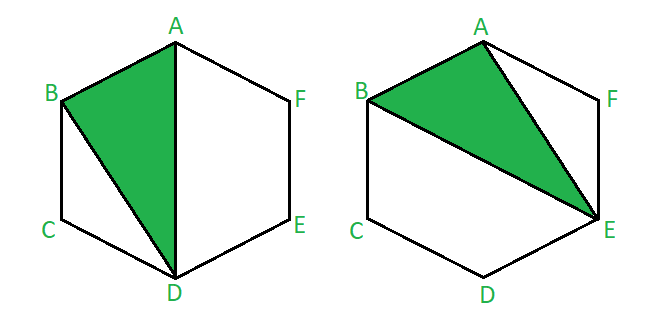Open in App
Not now

# Number of triangles formed by joining vertices of n-sided polygon with one side common

• Difficulty Level : Easy
• Last Updated : 20 Dec, 2022

Given N-sided polygon we need to find the number of triangles formed by joining the vertices of the given polygon with exactly one side being common.
Examples:

Input :
Output : 12
The image below is of a triangle forming inside a Hexagon by joining vertices as shown above. The two triangles formed has one side (AB) common with that of a polygon.It depicts that with one edge of a hexagon we can make two triangles with one side common. We can’t take C or F as our third vertex as it will make 2 sides common with the hexagon.Triangle with one side common of a hexagon

No of triangles formed ie equal to 12 as there are 6 edges in a hexagon.
Input :
Output :

Approach :

• To make a triangle with one side common with a polygon the two vertices adjacent to the chosen common vertices cannot be considered as the third vertex of a triangle.
• First select any one edge from the polygon. Consider this edge to be the common edge. Number of ways to select an edge in a polygon would be equal to n.
• Now ,to form a triangle ,select any of the (n-4) vertices left .Two vertices of the common edge and two vertices adjacent to the common edge cannot be considered.
• Number of triangle formed by joining the vertices of an n-sided polygon with one side common would be equal to n * ( n – 4) .

Below is the implementation of the above approach:

## C++

 `// C++ program to implement` `// the above problem` `#include ` `using` `namespace` `std;`   `// Function to find the number of triangles` `void` `findTriangles(``int` `n)` `{` `    ``int` `num;` `    ``num = n * (n - 4);`   `    ``// print the number of triangles` `    ``cout << num;` `}`   `// Driver code` `int` `main()` `{` `    ``// initialize the number of sides of a polygon` `    ``int` `n;` `    ``n = 6;`   `    ``findTriangles(n);`   `    ``return` `0;` `}`

## Java

 `// Java program to implement` `// the above problem` `import` `java.io.*;` `public` `class` `GFG` `{` `    `  `// Function to find the number of triangles` `static` `void` `findTriangles(``int` `n)` `{` `    ``int` `num;` `    ``num = n * (n - ``4``);`   `    ``// print the number of triangles` `    ``System.out.println(num);` `}`   `// Driver code` `public` `static` `void` `main(String [] args)` `{` `    ``// initialize the number of sides of a polygon` `    ``int` `n;` `    ``n = ``6``;`   `    ``findTriangles(n);`   `}` `}`   `// This code is contributed by ihritik`

## Python3

 `# Python3 program to implement` `# the above problem`   `# Function to find the number of triangles` `def` `findTriangles(n):` `    ``num ``=` `n ``*` `(n ``-` `4``)`   `    ``# print the number of triangles` `    ``print``(num)`   `# Driver code ` `# initialize the number of sides of a polygon` `n ``=` `6`   `findTriangles(n)`   `# This code is contributed by mohit kumar 29`

## C#

 `// C# program to implement` `// the above problem` `using` `System;`   `class` `GFG` `{` `    `  `// Function to find the number of triangles` `static` `void` `findTriangles(``int` `n)` `{` `    ``int` `num;` `    ``num = n * (n - 4);`   `    ``// print the number of triangles` `    ``Console.WriteLine(num);` `}`   `// Driver code` `public` `static` `void` `Main()` `{` `    ``// initialize the number of sides of a polygon` `    ``int` `n;` `    ``n = 6;`   `    ``findTriangles(n);`   `}` `}`   `// This code is contributed by ihritik`

## Javascript

 ``

Output:

`12`

Time Complexity: O(1)
Auxiliary Space: O(1)

My Personal Notes arrow_drop_up
Related Articles